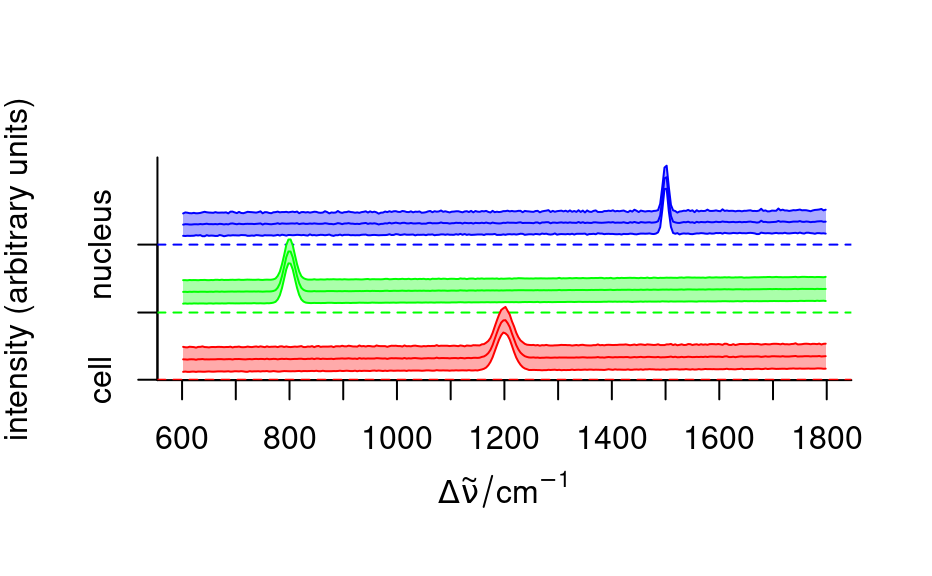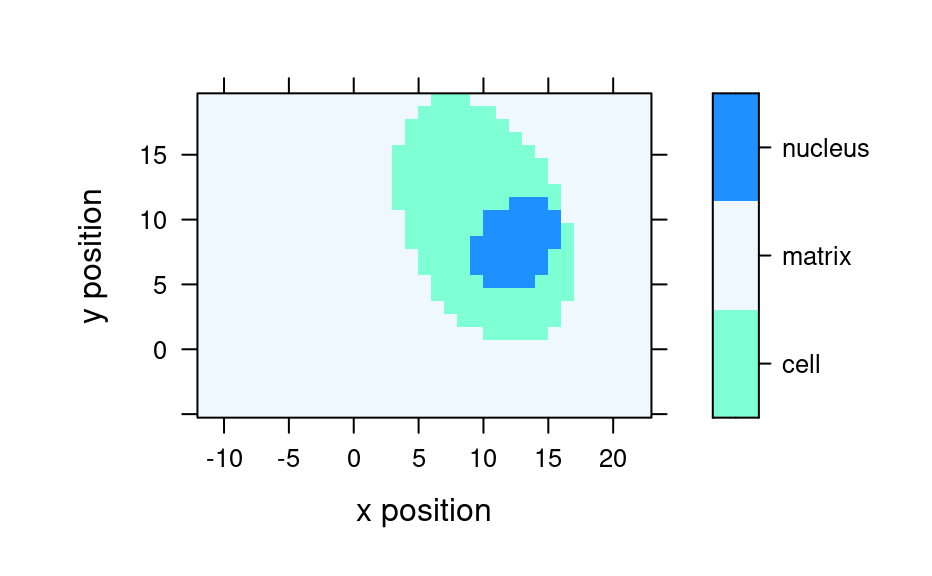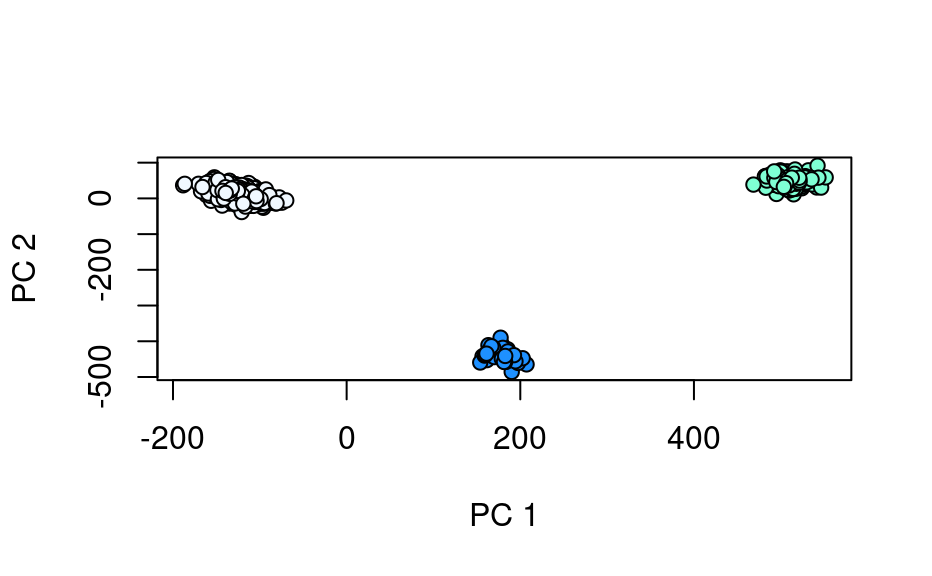This is a synthetic data set intended for testing and demonstration. Function generate_faux_cell() simulates the faux cell data (note: in the future, it is planned to parameterize the funcion) and object faux_cell is an instance of this dataset generated first time it is used.

The data set resembles the chondro data set but is entirely synthetic.

generate_faux_cell()

faux_cell

Format

The object faux_cell is a hyperSpec object that contains 875 Raman-like spectra, allocated to three groups/regions in column region: the matrix/background, the cell and the cell nucleus. Each spectrum is composed of 300 data points. The spectrum of each region is unique and simple, with a single peak at a particular frequency and line width. Poisson noise has been added. The data is indexed along the x and y dimensions, simulating data collected on a grid.

An object of class hyperSpec of dimension 875 x 4 x 300.

Author

Claudia Beleites, Bryan A. Hanson

Examples

set.seed(1)
faux_cell <- generate_faux_cell()

faux_cell
#> hyperSpec object
#>    875 spectra
#>    4 data columns
#>    300 data points / spectrum

plot(sample(faux_cell, 10), stacked = TRUE)# Plot mean spectra
fc_groups <- aggregate(faux_cell, faux_cell$region, mean_pm_sd) plot_spc(fc_groups, stacked = ".aggregate", col = c("red", "green", "blue"), fill = ".aggregate" )mapcols <- c(cell = "aquamarine", matrix = "aliceblue", nucleus = "dodgerblue") plotmap(faux_cell, region ~ x * y, col.regions = mapcols) #> Warning: device support for raster images unknown, ignoring 'raster=TRUE'# PCA pca <- prcomp(faux_cell) plot(pca)loadings <- decomposition(faux_cell, t(pca$rotation), scores = FALSE)plot(pca$x[, 2], pca$x[, 3],